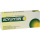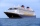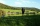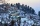# Integers + rational numbers - math problems

#### Number of problems found: 8

• BrianBrian divides 80 by 7. Between what two whole numbers is his answer?
• The elevationThe elevation of a sunken ship is -120 feet. Your elevation is 5/8 of the ship’s elevation. What is your elevation?
• A dolphinA dolphin was swimming 17 meters below the surface of the ocean. It located a squid and dove down 4 more meters to eat it. What is the location of the dolphin now relative to the surface?
• Find the 21Find the sum of the six terms of the finite geometric sequence 96, -48, 24, -12
• Cooler placeThe temperature recorded in Simla is -4°C and in Kufri is -6°C on the same day. Which place is cooler on the day?
• The temperatureThe temperature at 1:00 was 10 F. Between 1:00 and 2:00, the temperature dropped 15F. Between 2:00 and 3:00, the temperature rose 3F. What is the temperature at 3:00?
• Whole numbersPavol wrote down a number that is both rational and a whole number. What is one possible number she could have written down?
• FractionFraction frac(0, overline(38))(0,38) write as fraction a/b, a, b is integers numerator/denominator.

We apologize, but in this category are not a lot of examples.
Do you have an exciting math question or word problem that you can't solve? Ask a question or post a math problem, and we can try to solve it.

We will send a solution to your e-mail address. Solved examples are also published here. Please enter the e-mail correctly and check whether you don't have a full mailbox.

Integers - math problems. Rational numbers - math problems.